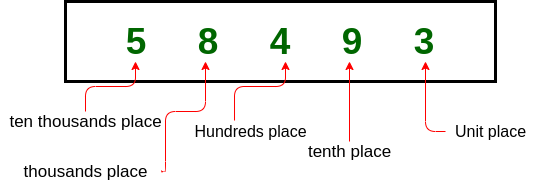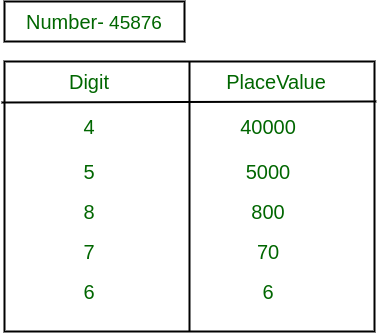# Place Value of a given digit in a number

Place value can be defined as the value represented by a digit in a number on the basis of its position in the number. Here is the illustration of place value with an example:Let’s take another example to illustrate place value for N = 45876Problem:
Given a positive integer N and a digit D. The task is to find out the place value of a digit D in the given number N. If multiple occurrences of digit occurs then find the minimum place value.

Example:

Input: N = 3928, D = 3
Output: 3000
Explaination:
Place value of 3 in this number is 3*1000 = 3000

Input: N = 67849, D = 6
Output: 60000

## Recommended: Please try your approach on {IDE} first, before moving on to the solution.

Solution Approach:

1. Find the position of the digit from the right side of the input number. In order to find the position take the mod of that number by 10 and check for the number and divide the number by 10.
2. When the position of the digit is found then just multiply digit with the 10 position.

Here is the implementation of the above approach:

## C++

 `// C++ implementation to find ` `// place value of a number ` `#include ` `using` `namespace` `std; ` ` `  `// Function to find place value ` `int` `placeValue(``int` `N, ``int` `num) ` `{ ` `    ``int` `total = 1, value = 0, rem = 0; ` `    ``while` `(``true``) ` `    ``{ ` `        ``rem = N % 10; ` `        ``N = N / 10; ` ` `  `        ``if` `(rem == num) ` `        ``{ ` `            ``value = total * rem; ` `            ``break``; ` `        ``} ` `        ``total = total * 10; ` `    ``} ` `    ``return` `value; ` `} ` ` `  `// Driver Code ` `int` `main() ` `{ ` ` `  `    ``// Digit, which we want ` `    ``// to find place value. ` `    ``int` `D = 5; ` ` `  `    ``// Number from where we ` `    ``// want to find place value. ` `    ``int` `N = 85932; ` ` `  `    ``cout << (placeValue(N, D)); ` `} ` ` `  `// This code is contributed by Ritik Bansal `

## Java

 `// Java implementation to find ` `// place value of a number ` ` `  `import` `java.util.*; ` `import` `java.io.*; ` `import` `java.lang.*; ` ` `  `class` `GFG { ` ` `  `    ``// function to find place value ` `    ``static` `int` `placeValue(``int` `N, ``int` `num) ` `    ``{ ` `        ``int` `total = ``1``, value = ``0``, rem = ``0``; ` `        ``while` `(``true``) { ` `            ``rem = N % ``10``; ` `            ``N = N / ``10``; ` ` `  `            ``if` `(rem == num) { ` `                ``value = total * rem; ` `                ``break``; ` `            ``} ` ` `  `            ``total = total * ``10``; ` `        ``} ` `        ``return` `value; ` `    ``} ` ` `  `    ``// Driver Code ` `    ``public` `static` `void` `main(String[] args) ` `    ``{ ` ` `  `        ``// Digit, which we want ` `        ``// to find place value. ` `        ``int` `D = ``5``; ` ` `  `        ``// Number from where we ` `        ``// want to find place value. ` `        ``int` `N = ``85932``; ` ` `  `        ``System.out.println(placeValue(N, D)); ` `    ``} ` `} `

## Python3

 `# Python3 implementation to find  ` `# place value of a number  ` ` `  `# Function to find place value  ` `def` `placeValue(N, num):  ` `     `  `    ``total ``=` `1` `    ``value ``=` `0` `    ``rem ``=` `0` `     `  `    ``while` `(``True``):  ` `        ``rem ``=` `N ``%` `10` `        ``N ``=` `N ``/``/` `10` ` `  `        ``if` `(rem ``=``=` `num):  ` `            ``value ``=` `total ``*` `rem  ` `            ``break` `        ``total ``=` `total ``*` `10` `         `  `    ``return` `value  ` ` `  `# Driver Code ` ` `  `# Digit, which we want  ` `# to find place value.  ` `D ``=` `5` ` `  `# Number from where we  ` `# want to find place value.  ` `N ``=` `85932` ` `  `print``(placeValue(N, D)) ` ` `  `# This code is contributed by divyamohan123  `

## C#

 `// C# implementation to find ` `// place value of a number ` `using` `System; ` `class` `GFG{ ` ` `  `// function to find place value ` `static` `int` `placeValue(``int` `N, ``int` `num) ` `{ ` `    ``int` `total = 1, value = 0, rem = 0; ` `    ``while` `(``true``)  ` `    ``{ ` `        ``rem = N % 10; ` `        ``N = N / 10; ` ` `  `        ``if` `(rem == num)  ` `        ``{ ` `            ``value = total * rem; ` `            ``break``; ` `        ``} ` ` `  `        ``total = total * 10; ` `    ``} ` `    ``return` `value; ` `} ` ` `  `// Driver Code ` `public` `static` `void` `Main() ` `{ ` ` `  `    ``// Digit, which we want ` `    ``// to find place value. ` `    ``int` `D = 5; ` ` `  `    ``// Number from where we ` `    ``// want to find place value. ` `    ``int` `N = 85932; ` ` `  `    ``Console.Write(placeValue(N, D)); ` `} ` `} ` ` `  `// This code is contributed by Code_Mech `

Output:

```5000
```

Time Complexity: O(log N)
Auxiliary space: O(1)

Attention reader! Don’t stop learning now. Get hold of all the important DSA concepts with the DSA Self Paced Course at a student-friendly price and become industry ready.

My Personal Notes arrow_drop_upCheck out this Author's contributed articles.

If you like GeeksforGeeks and would like to contribute, you can also write an article using contribute.geeksforgeeks.org or mail your article to contribute@geeksforgeeks.org. See your article appearing on the GeeksforGeeks main page and help other Geeks.

Please Improve this article if you find anything incorrect by clicking on the "Improve Article" button below.